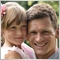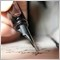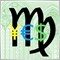# Why are the indicators (lines, arrows, histograms) flickering in the MT5 terminal? - page 280341

Try printing prev_calculated at the very beginning of OnCalculate.

And compare with some standard indicator - whether it flashes.915

Andrey Khatimlianskii: Try printing prev_calculated at the very beginning of OnCalculate. And compare it to some standard indicator - is it flashing.
Added two static variables to OnCalculate() function to compare current values with previous values:

static int last_rates_total=0; // previous value "input timeseries size at previous
static int last_prev_calculated=0; // previous value "bars processed at the previous call

and verification code with the output to the journal

```//+------------------------------------------------------------------+
//| Custom indicator iteration function
//+------------------------------------------------------------------+
int OnCalculate (const int rates_total,      // размер входных таймсерий
const int prev_calculated,  // обработано баров на предыдущем вызове
...

{
int N=rates_total-prev_calculated; if (N>MAX_PERIOD) N-=MAX_PERIOD;

static int last_rates_total=0;     // размер входных таймсерий
static int last_prev_calculated=0; // обработано баров на предыдущем вызове
if (rates_total!=last_rates_total || prev_calculated!=last_prev_calculated) {
Print("N=", N,  ", rates_total=", rates_total, ", prev_calculated=", prev_calculated);
last_rates_total=rates_total; last_prev_calculated=prev_calculated;
}

for (int i=N; i>0 && !IsStopped(); i--) {
...
}
return(rates_total);
}
```

Started the AOM indicator on the minute and got this report

```FH      0       12:38:02.271    AOM (Si-6.17,M1)        N=41934, rates_total=41969, prev_calculated=0
FN      0       12:38:02.275    AOM (Si-6.17,M1)        N=0, rates_total=41969, prev_calculated=41969
DE      0       12:38:51.845    AOM (Si-6.17,M1)        N=1, rates_total=41970, prev_calculated=41969
GH      0       12:38:51.845    AOM (Si-6.17,M1)        N=0, rates_total=41970, prev_calculated=41970
PO      0       12:39:51.207    AOM (Si-6.17,M1)        N=1, rates_total=41971, prev_calculated=41970
EE      0       12:39:51.215    AOM (Si-6.17,M1)        N=0, rates_total=41971, prev_calculated=41971
MH      0       12:40:52.423    AOM (Si-6.17,M1)        N=1, rates_total=41972, prev_calculated=41971
IO      0       12:40:52.700    AOM (Si-6.17,M1)        N=0, rates_total=41972, prev_calculated=41972
CR      0       12:41:51.214    AOM (Si-6.17,M1)        N=1, rates_total=41973, prev_calculated=41972
OH      0       12:41:51.214    AOM (Si-6.17,M1)        N=0, rates_total=41973, prev_calculated=41973
RO      0       12:42:51.207    AOM (Si-6.17,M1)        N=1, rates_total=41974, prev_calculated=41973
HR      0       12:42:51.207    AOM (Si-6.17,M1)        N=0, rates_total=41974, prev_calculated=41974
NI      0       12:43:51.439    AOM (Si-6.17,M1)        N=1, rates_total=41975, prev_calculated=41974
RO      0       12:43:51.446    AOM (Si-6.17,M1)        N=0, rates_total=41975, prev_calculated=41975
RR      0       12:44:51.373    AOM (Si-6.17,M1)        N=1, rates_total=41976, prev_calculated=41975
DI      0       12:44:51.373    AOM (Si-6.17,M1)        N=0, rates_total=41976, prev_calculated=41976
ML      0       12:45:51.449    AOM (Si-6.17,M1)        N=1, rates_total=41977, prev_calculated=41976
GR      0       12:45:51.902    AOM (Si-6.17,M1)        N=0, rates_total=41977, prev_calculated=41977
II      0       12:46:51.541    AOM (Si-6.17,M1)        N=1, rates_total=41978, prev_calculated=41977
GL      0       12:46:51.541    AOM (Si-6.17,M1)        N=0, rates_total=41978, prev_calculated=41978
PS      0       12:47:51.286    AOM (Si-6.17,M1)        N=1, rates_total=41979, prev_calculated=41978
PI      0       12:47:51.303    AOM (Si-6.17,M1)        N=0, rates_total=41979, prev_calculated=41979
QL      0       12:48:51.395    AOM (Si-6.17,M1)        N=1, rates_total=41980, prev_calculated=41979
IS      0       12:48:51.408    AOM (Si-6.17,M1)        N=0, rates_total=41980, prev_calculated=41980
DF      0       12:49:51.790    AOM (Si-6.17,M1)        N=1, rates_total=41981, prev_calculated=41980
PL      0       12:49:51.790    AOM (Si-6.17,M1)        N=0, rates_total=41981, prev_calculated=41981
```

Nothing unexpected, normal processing of only new bars coming in is going on.80341

Eugene Myzrov:
Added two static variables to OnCalculate() function to compare current values with previous values:

Nothing unexpected, there is a normal process of handling only new bars coming in.

Are the indicators from the standard delivery flashing?915

Andrey Khatimlianskii: Are the indicators from the standard delivery blinking?

No, I put a couple of standard indicators "Moving Average", "Awesome Oscillator" on the chart. Observed. No, I did not notice any flickering or disappearing values in"Data Window".80341

Eugene Myzrov:

No, I put a couple of standard indicators "Moving Average", "Awesome Oscillator" on the chart. Observed. No, I did not notice any flickering or disappearance of values in"Data Window".

I mean those indicators that are in the source (mq5).

If they do not blink, then the problem is obviously in your code. Simplify it until it stops flickering. Or vice versa - refine the standard code to your state, until it flickers.39986

This is a MT5 feature, imho.915

Stanislav Korotky: It's a MT5 feature, imho.

So you have to accept it, take it for granted and stop looking for the cause?915

Andrey Khatimlianskii: I mean those indicators which are in the source code (mq5). If they do not blink, then the problem is obviously in your code. Simplify it until it stops flickering. Or vice versa - refine the standard code to your state, until it flickers.
But I've already simplified the code to the point of flicker. Ok, I'll try to go the opposite way.

By the way, I haven't found a universal "wrapper" for OnCalculate() function, even among standard indicators, to use it once and for all.
Maybe, you know some indicator that has such a "wrapper" to use it as a reference?39986

Eugene Myzrov:

So you have to accept it, take it for granted and stop looking for a reason?

Write to the service desk.80341

Eugene Myzrov:
But I've already simplified the code to the extreme. Ok, I'll try to go in other way.

It may be about any little thing.

Eugene Myzrov:

By the way, I have not yet found a universal "wrapper" for the OnCalculate() function, even among the standard indicators, to use it once and for all.
Maybe, you may suggest an indicator which has such a "wrapper" to use it as a reference?

I have seen several variants. You may search and choose.
I use this one myself:

```        //---
if ( rates_total < MinBars ) return(0);

//--- last counted bar will be recounted
int limit=rates_total-prev_calculated-1;
if(prev_calculated>0) limit++;

//---
for( int i=limit; i>=0; i--)
{